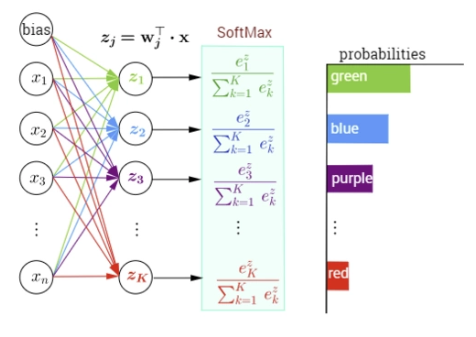# 一文搞懂交叉熵损失

### 从熵来看交叉熵损失

#### 信息量

$$X$$是一个离散型随机变量，其取值为集合$$X = {x_0,x_1,\dots,x_n}$$ ，则其概率分布函数为$$p(x) = Pr(X = x),x \in X$$，则定义事件$$X = x_0$$ 的信息量为：

$I(x_0) = -\log(p(x_0))$

$$p(x_0) = 1$$时，该事件必定发生，其信息量为0.

#### 熵

$H(x) = -\sum_{i=1}^np(x_i)\log(p(x_i))$

#### 相对熵 Relative entropy

$$p(x),q(x)$$ 分别是 离散随机变量$$X$$的两个概率分布，则$$p$$$$q$$的相对熵是：

$D_{KL}(p \parallel q) = \sum_i p(x_i) log(\frac{p(x_i)}{q(x_i)})$

• 如果$$p(x)$$$$q(x)$$的分布相同，则其相对熵等于0
• $$D_{KL}(p \parallel q) \neq D_{KL}(q \parallel p)$$，也就是相对熵不具有对称性。
• $D_{KL}(p \parallel q) \geq 0$

#### 交叉熵 Cross Entropy

$$p(x),q(x)$$ 分别是 离散随机变量$$X$$的两个概率分布，其中$$p(x)$$是目标分布，$$p$$$$q$$的交叉熵可以看做是，使用分布$$q(x)$$ 表示目标分布$$p(x)$$的困难程度：

$H(p,q) = \sum_ip(x_i)log\frac{1}{\log q(x_i)} = -\sum_ip(x_i)\log q(x_i)$

\begin{align} H(p) &= -\sum_{i}p(x_i) \log p(x_i) \\ D_{KL}(p \parallel q) &= \sum_{i}p(x_i)\log \frac{p(x_i)}{q(x_i)} = \sum_i (p(x_i)\log p(x_i) - p(x_i) \log q(x_i)) \\ H(p,q) &= -\sum_ip(x_i)\log q(x_i) \end{align}

$D_{KL}(p,q) = H(p,q)- H(p)$

$$p(x)$$ 是目标分布（训练数据的分布），我们的目标的就让训练得到的分布$$q(x)$$尽可能的接近$$p(x)$$，这时候就可以最小化$$D_{KL}(p \parallel q)$$，等价于最小化交叉熵$$H(p,q)$$

### 从最大似然看交叉熵

$L(\theta) = q(X; \theta) = \prod_i^mq(x_i;\theta)$

$\theta_{ML} = arg \max_{\theta} \prod_i^mq(x_i;\theta)$

$\theta_{ML} = arg \max_\theta \sum_i^m \log q(x_i;\theta)$

$\theta_{ML} = arg \max_\theta \frac{1}{m}\sum_i^m\log q(x_i;\theta)$

#### 和相对熵等价

$\frac{1}{m}\sum_i^m\log q(x_i;\theta)$

$\frac{1}{m}\sum_i^m \log q(x_i;\theta) \rightarrow E_{x\sim P}(\log q(x;\theta))$

\begin{aligned} \theta_{ML} &= arg \max_{\theta} E_{x\sim P}({\log q(x;\theta)}) \\ &= arg \min_{\theta} E_{x \sim P}(- \log q(x;\theta)) \end{aligned}

\begin{aligned} D_{KL}(p \parallel q) &= \sum_i p(x_i) log(\frac{p(x_i)}{q(x_i)})\\ &= E_{x\sim p}(\log \frac{p(x)}{q(x)}) \\ &= E_{x \sim p}(\log p(x) - \log q(x)) \\ &= E_{x \sim p}(\log p(x)) - E_{x \sim p} (\log q(x)) \end{aligned}

#### 和交叉熵等价

\begin{aligned}\theta_{ML} &= -arg \min_\theta E_{x\sim p}\log q(x;\theta) \\D_{KL} &= E_{x \sim p}\log p(x) - E_{x \sim p} \log q(x) \\H(p,q) &= -\sum_i^m p(x_i) \log q(x_i) = -E_{x \sim p} \log q(x)\end{aligned}\begin{aligned}\theta_{ML} &= arg \min_\theta E_{x\sim p}\log q(x;\theta) \\D_{KL} &= E_{x \sim p}\log p(x) - E_{x \sim p} \log q(x) \\H(p,q) &= -\sum_i^m p(x_i) \log q(x_i) = -E_{x \sim p} \log q(x)\end{aligned}

### 多分类交叉熵

softmax函数定义如下：

$S_i = \frac{e^{z_i}}{\sum^n_{i=1}e^{z_i}}$#### Cross Entropy Loss

$q(c_i) = \frac{e^{z_i}}{\sum^n_{i=1}e^{z_i}}$

$H(p,q) =-\sum_ip(c_i)\log q(c_i)$

\begin{align} p(cat) & = 1 \\ p(pig) &= 0 \\ p(dog) & = 0 \end{align}

\begin{aligned} H(p,q) &= -(p(cat) \log q(cat) + p(pig) + \log q(pig) + \log q(dog)) \\ &= - (1 \cdot \log 0.6 + 0 \cdot \log 0.2 +0 \cdot \log 0.2) \\ &= - \log 0.6 \\ &= - \log q(cat) \end{aligned}

\begin{aligned} \text{cat} &= (1 0 0) \\ \text{pig} &= (010) \\ \text{dog} &= (001) \end{aligned}

$\text{Cross_Entropy}(p,q) = - \log q(c_i)$

#### PyTorch中的Cross Entropy

PyTorch中实现交叉熵损失的有三个函数torch.nn.CrossEntropyLosstorch.nn.LogSoftmax以及torch.nn.NLLLoss

• torch.nn.functional.log_softmax 比较简单，输入为$$n$$维向量，指定要计算的维度dim，输出为$$log(Softmax(x))$$。其计算公式如下：

$\text{LogSoftmax}(x_i) = \log (\frac{\exp(x_i)}{\sum_j \exp(x_j)})$

• torch.nn.functional.nll_loss 负对数似然损失（Negative Log Likelihood Loss)，用于多分类，其输入的通常是torch.nn.functional.log_softmax的输出值。其函数如下
torch.nn.functional.nll_loss(input, target, weight=None, size_average=None, ignore_index=-100, reduce=None, reduction='mean')


input 也就是log_softmax的输出值，各个类别的对数概率。target 目标正确类别,weight 针对类别不平衡问题，可以为类别设置不同的权值；ignore_index 要忽略的类别，不参与loss的计算；比较重要的是reduction 的值，有三个取值：none 不做处理，输出的结果为向量；meannone结果求均值后输出；sumnone 结果求和后输出。

• torch.nn.CrossEntropyLoss就是上面两个函数的组合nll_loss(log_softmax(input))

### 二分类交叉熵

$$\text{sigmoid}$$ 函数的表达式如下：

$\sigma(z) = \frac{1}{1 + e^{-z}}$

sigmoid的输入为$$z$$ ，其输出为$$(0,1)$$ ，可以表示分类为正样本的概率。

$\text{Cross_Entropy}(p,q) = -\sum_i^m p(x_i) \log q(x_i)$

\begin{aligned} \text{Cross_Entropy}(p,q) &= -(p(x_1) \log q(x_1) + p(x_2) \log q(x_2)) \end{aligned}

$\text{Cross_Entropy}(p,q) = -(p \log q + (1-p) \log (1-q))$

### 总结

$H(p,q)= D_{KL}(p \parallel q) + H(p)$

$\theta_{ML} = -arg \min_\theta E_{x\sim p}\log q(x;\theta)$

posted @ 2019-12-19 15:29  Brook_icv  阅读(43235)  评论(3编辑  收藏  举报